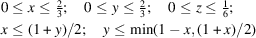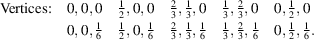International
Tables for
Crystallography
Volume A
Space-group symmetry
Edited by M. I. Aroyo

International Tables for Crystallography (2016). Vol. A, ch. 2.1, pp. 159-160

## Section 2.1.3.8. Asymmetric unit

Th. Hahna and A. Looijenga-Vosb

#### 2.1.3.8. Asymmetric unit

| top | pdf |

An asymmetric unit of a space group is a (simply connected) smallest closed part of space from which, by application of all symmetry operations of the space group, the whole of space is filled. This implies that mirror planes and rotation axes must form boundary planes and boundary edges of the asymmetric unit. A twofold rotation axis may bisect a boundary plane. Centres of inversion must either form vertices of the asymmetric unit or be located at the midpoints of boundary planes or boundary edges. For glide planes and screw axes, these simple restrictions do not hold. An asymmetric unit contains all the information necessary for the complete description of the crystal structure. In mathematics, an asymmetric unit is called fundamental region' or fundamental domain'.

#### Example

The boundary planes of the asymmetric unit in space group Pmmm (47) are fixed by the six mirror planes x, y, 0;; x, 0, z;; 0, y, z; and. For space group(19), on the other hand, a large number of connected regions, each with a volume of(cell), may be chosen as asymmetric unit.

In cases where the asymmetric unit is not uniquely determined by symmetry, its choice may depend on the purpose of its application. For the description of the structures of molecular crystals, for instance, it is advantageous to select asymmetric units that contain one or more complete molecules. In the space-group tables of this volume, following IT A (2002), the asymmetric units are chosen in such a way that Fourier summations can be performed conveniently.

For all triclinic, monoclinic and orthorhombic space groups, the asymmetric unit is chosen as a parallelepiped with one vertex at the origin of the cell and with boundary planes parallel to the faces of the cell. It is given by the notationwherestands for x, y or z.

For space groups with higher symmetry, cases occur where the origin does not coincide with a vertex of the asymmetric unit or where not all boundary planes of the asymmetric unit are parallel to those of the cell. In all these cases, parallelepipedsare given that are equal to or larger than the asymmetric unit. Where necessary, the boundary planes lying within these parallelepipeds are given by additional inequalities, such as,etc.

In the trigonal, hexagonal and especially the cubic crystal systems, the asymmetric units have complicated shapes. For this reason, they are also specified by the coordinates of their vertices. Drawings of asymmetric units for cubic space groups have been published by Koch & Fischer (1974). Fig. 2.1.3.11shows the boundary planes occurring in the tetragonal, trigonal and hexagonal systems, together with their algebraic equations.Figure 2.1.3.11 | top | pdf |Boundary planes of asymmetric units occurring in the space-group tables. (a) Tetragonal system. (b) Trigonal and hexagonal systems. The point coordinates refer to the vertices in the plane.

#### Examples

 (1) In space group P4mm (99), the boundary planeoccurs in addition to planes parallel to the unit-cell faces; the asymmetric unit is given by(2) In P4bm (100), one of the boundary planes is. The asymmetric unit is given by(3) In space group R32155; hexagonal axes, the boundary planes are, among others,,,. The asymmetric unit is defined byIt is obvious that the indication of the vertices is of great help in drawing the asymmetric unit.

Fourier syntheses. For complicated space groups, the easiest way to calculate Fourier syntheses is to consider the parallelepiped listed, without taking into account the additional boundary planes of the asymmetric unit. These planes should be drawn afterwards in the Fourier synthesis. For the computation of integrated properties from Fourier syntheses, such as the number of electrons for parts of the structure, the values at the boundaries of the asymmetric unit must be applied with a reduced weight if the property is to be obtained as the product of the content of the asymmetric unit and the multiplicity.

#### Example

In the parallelepiped of space group Pmmm (47), the weights for boundary planes, edges and vertices are,and, respectively.

Asymmetric units of the plane groups have been discussed by Buerger (1949, 1960) in connection with Fourier summations.

### References

International Tables for Crystallography (2002). Vol. A, 5th ed., edited by Th. Hahn. Dordrecht: Kluwer Academic Publishers. [Abbreviated as IT A (2002).]
Buerger, M. J. (1949). Fourier summations for symmetrical crystals. Am. Mineral. 34, 771–788.
Buerger, M. J. (1960). Crystal-Structure Analysis, ch. 17. New York: Wiley.
Koch, E. & Fischer, W. (1974). Zur Bestimmung asymmetrischer Einheiten kubischer Raumgruppen mit Hilfe von Wirkungsbereichen. Acta Cryst. A30, 490–496.